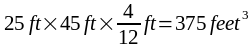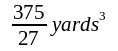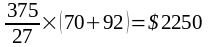SEARCH HOMEMath Central Quandaries & QueriesQuestion from arlene, a parent: What is the total cost of a driveway that is 25' wide and 45' long with 4" thickness if the concreat cost 92.00 per cubic yard and labor is 70.00 a cubic yard?Hi Arlene.

Cubic yards are a volume measurement, so you need to convert the driveway into a volume measurement as well in order to proceed to the cost.

Treat the driveway like a big flat box. First convert the the length measurements to compatible units. Since two measurements are in feet, I can convert the thickness from inches to feet by dividing it by twelve, then I can multiply length times width times depth and get a volume measurement.You see that because I multiplied feet times feet times feet, my result is in feet^3 (cubic feet). But my cost rates are based on cubic yards, so I have to convert. To convert feet to yards, I divide by 3. Therefore to convert from feet^3 to yards^3, I divide by 3^3, and 3^3 = 27. So I divide by 27:Finally, I have to multiply by the cost per cubic yard to get the total bill.Cheers,
Stephen La RocqueMath Central is supported by the University of Regina and The Pacific Institute for the Mathematical Sciences.